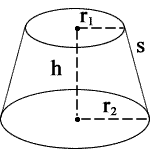## Conical frustum calculator

 r1 = r2 = h = Units: s = 0 V = 0 L = 0 T = 0 B = 0 A = 0
» Show Work

r1 = radius 1, r2 = radius 2, h = height, s = slant height, V = volume

L = lateral surface area, T = top surface area, B = base surface area,A = total surface area

A frustum formingan upper base and a lower base that are circular and parallel, It can be formed from a right circular cone by cutting off the tip of the cone.For example, To r1 = 2, r2 = 3, h = 4,

1. To calculate s, use the following formula: √((r1 - r2)2 + h2). When you substitute r1, r2, and h for their values, you get √((2 - 3)2 + 42), which equals 4.12311 m.
2. To calculate V, use the following formula: (1/3)πh(r12 + r22 + (r1 + r2)). When you substitute r1, r2, and h for their values, you get (1/3)π*4*(22 + 32 + (2 * 3)), which equals 79.58701 m3.
3. To calculate L, use the following formula: π * (r1 + r2) * s. When you substitute r1, r2, and s for their values, you get π * (2 + 3) * 4.12311, which equals 64.76566 m2.
4. To calculate T, use the following formula: πr12. When you substitute r1 for its value, you get π * 22, which equals 12.56637 m2.
5. To calculate B, use the following formula: πr22. When you substitute r2 for its value, you get π * 32, which equals 28.27433 m2.
6. To calculate A, use the following formula: π(r12 + r22 + (r1 + r2) * s). When you substitute r1, r2, and s for their values, you get π(22 + 32 + (2 + 3) * 4.12311), which equals 105.60636 m2.

Thinkcalculator.com provides you helpful and handy calculator resources.